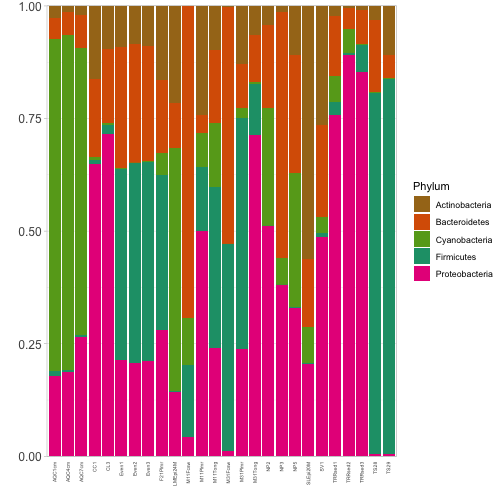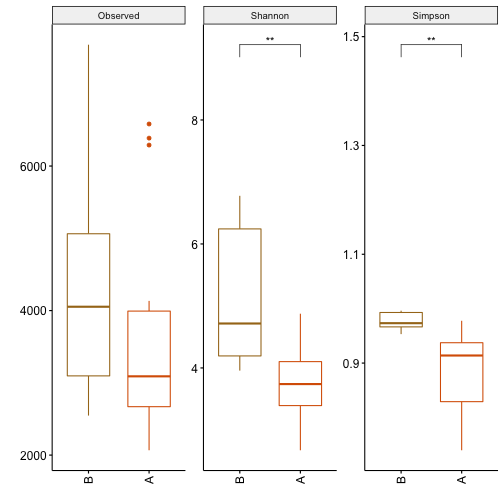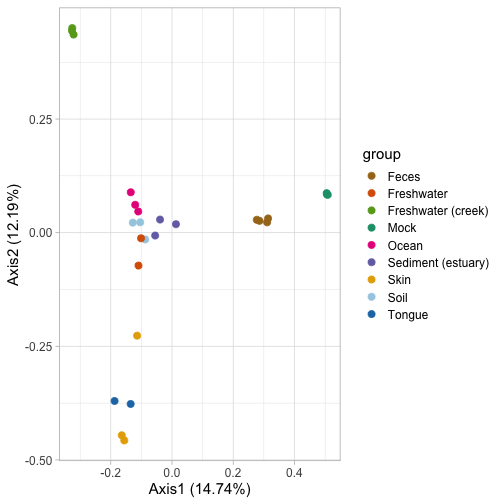microbial is a R package for microbial community analysis with dada2 (https://benjjneb.github.io/dada2/) and phyloseq (https://joey711.github.io/phyloseq/). microbial package is developed to enhance the available statistical analysis procedures in R by providing simple functions to analysis and visualize the 16S rRNA data.Here we present a tutorial with minimum working examples to demonstrate usage and dependencies.

## 1. Data format/ requirement

To use the package user can start with the raw fastq files with sample information ready or user can start with a phyloseq object (phloseq-class) comprising taxa abundance information, taxonomy assignment, sample data which is a combination of the measured environmental variables and any categorical variables present in the sample.

If the phylogenetic tree is available, it can also be part of it, but it has nothing to do with most of the functions implemented here so far. We chose to use this format because as the analysis and visualization proceed, we have many options to process the data.User can go to the https://github.com/joey711/phyloseq to check the detail format of phyloseq object.

## 2 Example data

The physeq data were the Global patterns of 16S rRNA diversity at a depth of millions of sequences per sample (2011). The data was published in PNAS in early 2011. This work compared the microbial communities from 25 environmental samples and three known “mock communities” – a total of 9 sample types – at a depth averaging 3.1 million reads per sample. Authors were able to reproduce diversity patterns seen in many other published studies, while also invesitigating technical issues/bias by applying the same techniques to simulated microbial communities of known composition. We simple modified the data with add one more group factor to display some functions implemented in the package.

## 3. Software Usage

### 3.1 Installation

Install the package with its dependencies and load it for usage in R.

``````library(devtools) # Load the devtools package
install_github("guokai8/microbial") # Install the package
``````

### 3.2 Start from fastq files

To start with the raw fastq files, the users need first have the reference database ready. The preRef function provides easy way to download the references. The ref_db parameter is a character to choose which database to download: silva, rdp and Greengenes. The path parameter show the location to store the database.

``````preRef(ref_db = "silva", path=".")
``````

Then users can use the plotquality function to show the quality of the reads which will help the users to specify processSeq to perform the analysis with dada2 package. The parameters included in this function were same as the dada2 function. The path is the working path for the input reads. The truncLen is an optional parameter with the default 0 (no truncation): Truncate reads after truncLen bases. Reads shorter than this are discarded. The trimLeft and trimRight are the number of nucleotides to remove from the start and end of each read.

``````?processSeq
``````

### 3.3 Data normalisation

Microbial community data is mainly OTU abundance (counts) with different design data.It is usually necessary that data is transformed by a suitable normalisation method. We provided different methods including; “relative”, “TMM”,variance stabilisation “vst” and “log2” for normalisation of taxa abundance. The function takes a phyloseq object physeq and returns a similar object whose otu-table component is normalised by a selected method as shown in the following examples.

``````library(microbial)
data("Physeq")
#default normalize method is relative
phy <- normalize(physeq, method = "relative")
``````

### 3.4 Relative abundance among all samples or groups

We first use relative normalised bacteria abundance to obtain the proportion of per sample. Then we generate the figure to show the proportion among all samples based on “Phylum” level. The group parameter is provided to show the proportion based on group level.

``````plotbar(phy,level="Phylum")
````````````#or among two group
# plotbar(phy,level="Phylum", group="group")
``````

### 3.5 Alpha diversity with wilcoxon test or t-test

The richness calculate the alpha diversity of provided community data using selected indices/method(s). Alpha diversity refers to the diversity within a particular area or ecosystem, and is usually expressed by the number of species. The plotalpha function performs pair-wise wilcoxon test or t-test of diversity measures between groups and outputs a plot for each of the selected methods(indices) annotated with significance labels.

The method in the richness function include: “Observed”, “Chao1”, “ACE”, “Richness”, “Fisher”, “Simpson”, “Shannon”, “Evenness” and “InvSimpson”. The group paramater in the plotalpha function is a categorical variable for which the grouping should be based on during the analysi, the group should be one of the sample_data column. pvalue specifies the p-value threshold for significance in wilcoxon, default is set to 0.05. User can also choose to use the padj paramter instead of pvalue. The plotalpha function return a ggplot2 object which will easy to modified by user.

``````plotalpha(physeq, group = "group")
``````### 3.6 Beta diversity

Beta diversity is a comparison of of diversity between groups, usually measured as the amount of species change between the groups. In the example provided below, we first normalize the taxa abundance to relative abundance to obtain the proportion of most abundant taxa per sample. The arguments in the plotbeta function include: physeq which a required phyloseq object, the distance which is a dissimilarity distance measure with otions of “bray” (default), “unifrac”,“wunifrac”,“manhattan”, “euclidean”, “canberra”, “bray”, “kulczynski”, “jaccard” and other distance methods, the group is a character string specifying a variable whose levels are the groups in the data, the method paramater is a character string specifying ordination method. All methods available to the ordinate function of phyloseq are acceptable here as well. The plotbeta function will return ggplot2 object.

``````plotbeta(phy, group="SampleType")
``````We also provide betatest function by doing permutation analysis of variance (PERMANOVA) and return corresponding r-squared and p-values, beta dispersion between all posible pairwise combinations of levels in the grouping variable is calculated and results return as a table.

``````beta <-betatest(phy,group="SampleType")
``````

### 3.7 Differentail expression

Here we provide difftest function to find features that are up or down regulated in the compared groups using DESeq2 package. The plotdiff function produce figure of the top most features annotated with corresponding adjusted p-values and abundance distribution. The difftest require a phyloseq object containing merged information of abundance, taxonomic assignment, sample data including the measured variables and categorical information of the samples. Raw count values are preferred for this function. The group paramater is a character string specifying the name of a categorical variable containing grouping information. The pvalue and log2FC are thresholds for p values and log2 fold change. Adjusted p value cutoff is also supported by specify the padj paramater.

``````res <- difftest(physeq,group="group")
``````

The plotdiff function require the differential test results from difftest. And the _level parameter provide which level to show: “Genus”, “Species” or other level. Other paramaters can be found in the man page of plotdiff.

``````plotdiff(res,level="Genus",padj=0.001,log2FC = 7,fontsize.y = 3)
``````

### 3.8 Biomarker selection

In addition we implement classification using random forest classifier and LEfSe method to find most import features among the groups. Random forests or random decision forests are an ensemble learning method for classification. And the random forest classifier is used to determine the importance of differentially expressed bacteria/taxa to the microbial community. Typically, we will use the Mean Descrease in Accuracy to measure the importance for each bacteria/taxa. The biomarker function do the random forest classification and return the sigificant table include the importance values. Raw count values are preferred for this function, and user can specify the normalize method with the method parameter.

``````res <- biomarker(physeq,group="group",ntree = 100)
``````

The plotmarker function will generate the figures based on the biomarker result. User can specify level to show with the level parameter and also the top parameter will choose the number of top most importance bacteria and taxa to draw.

``````plotmarker(res,level="Genus")
``````

We also provide ldamarker function to do the LEfSe analysis which base on the kruskal-wallis test and the LDA analysis. The parameters include physeq (A phyloseq object) and group (a character string specifying the name of a categorical variable containing grouping information. ). Raw count values are preferred for this function, and user can also specify the normalize method with the method parameter.

``````res <- ldamarker(physeq,group="group")
``````

The plotLDA function take the results from ldamarker and group factor which was used for the LEfSe analysis to generate figure with the significant bacteria marker.

``````plotLDA(res,group=c("A","B"),lda=5,pvalue=0.05)
``````

## 4. Dependencies

This packages depends on a number of other packages which include: phyloseq, vegan, DESeq2, ggplot2,randomForest. The package is still under development. New functions will be provided soon.

## 5. Contact information

For any questions please contact guokai8@gmail.com or submit the issues to https://github.com/guokai8/microbial/issues